How to Combine IF AND Formulas in Excel - Compute Expert

# How to Combine IF AND Formulas in Excel

Home >> Excel Tutorials from Compute Expert >> Excel Formulas List >> How to Combine IF AND Formulas in Excel

In this tutorial, you will learn how to combine IF AND formulas in excel completely. We sometimes combine these formulas if we need to evaluate multiple logic conditions to get our data processing result.

Before learning about the combination, it will be better if you have got the basics of each formula first. You can go to this link to learn about IF and this link to learn about AND if you need to.

Disclaimer: This post may contain affiliate links from which we earn commission from qualifying purchases/actions at no additional cost for you. Learn more

## The Way to Write It and Its Inputs

How to combine IF and AND in one formula writing in excel? Here is the general writing form of these two formulas combination.

= IF ( AND ( logic_condition1 , logic_condition2 , … ) , result_if_true , result_if_false )

We write AND as the input of our IF and input the logic conditions we need to evaluate to this AND. We usually do this if we need to evaluate multiple logic conditions to get our IF result. The evaluation result, of course, will follow the AND formula logic.

The AND formula logic is to give us TRUE if all of its logic condition inputs are TRUE. If at least one of them is FALSE, then AND will give us FALSE.

From the AND result, our IF will produce its final result accordingly. It will produce its true result if AND produces TRUE and it will produce its false result if AND produces FALSE. Therefore, we just need to input the true and false results of our IF as usual after we write our AND.

## Example of Its Usage and Result

Below is the implementation example of the IF and AND combination in excel.In this example, we have the condition where we have to evaluate three test scores. If at least one of them fails, then we regard the overall assessment result as a “fail”. If all of them pass, then we regard the overall assessment as a “pass”.

When we need to do this kind of data processing, we can combine IF and AND to help us.

Just input all the test score passing conditions to the AND that we write here. As all the test scores need to be above 70, we write the logic conditions that do that comparison. You can see how we do that in the example screenshot.

After we write our AND, we input the true and false results of our IF. As if AND produces TRUE means the overall assessment is a “pass”, we input “pass” for the IF true result here. For the IF false result, we input “fail”.

We do all that and we get all the assessment results we need from the test score groups that we have!

## Writing Steps

Need to know the way to write the IF and AND formulas combination in excel from the beginning? Learn from its writing steps we detail for you below!

1. Type an equal sign ( = ) in the cell where you want to put your IF AND result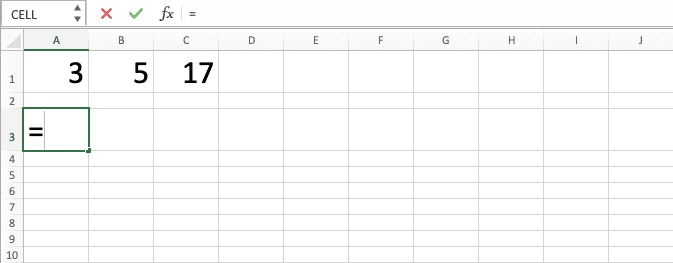2. Type IF (can be with large and small letters) and an open bracket sign after =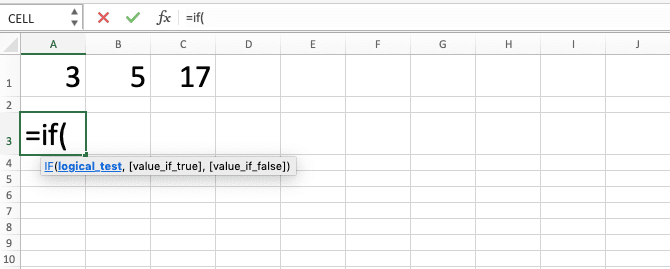3. Type AND (can be with large and small letters) and an open bracket sign4. Input all the logic conditions you want to process. Separate them with comma signs ( , )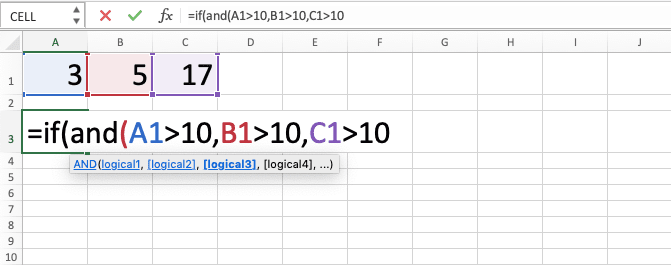5. Type a close bracket sign if you have inputted all the logic conditions you want to process. Then, type a comma sign6. Input the IF result you want if all the logic conditions you input previously are true. Then, type a comma sign7. Input the IF result you want if at least one of your logic conditions is false8. Type a close bracket sign9. Press Enter
10. Done!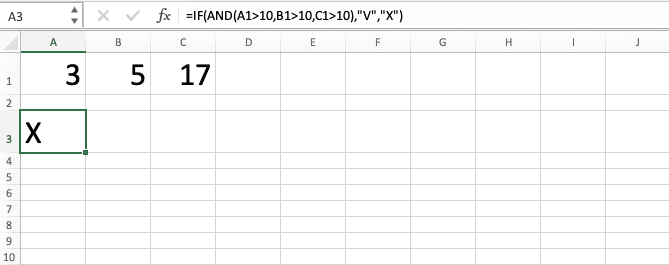## IF AND to Catch a Number in a Range

After you have learned the basics of IF AND combination in excel, now let’s learn a bit about its application. One of its most popular applications is to get a result if our number is in a range.

As things stand, you cannot seem to input a logic condition where a number is in a range. For this, you need to separate the range into two logic conditions and this is where IF AND can help.

The two logic conditions here are when our number is within the upper limit and lower limit of the range. We input the two logic conditions into AND to produce a result if our number is within this range.

Here is the general writing form of IF AND that summarizes that line of thinking.

= IF ( AND ( number < range_upper_limit , number > range_lower_limit ) , result_if_true , result_if_false )

As we want our number to be within range to produce our IF true result, we input logic conditions like that. If our number is below the range upper limit and above the range lower limit, it means it is within the range. Thus, we input these logic conditions in which we compare our number with the range limits to AND.

You can replace the < and > with <= and >= if you also have an “equal to” condition for the range.

Here is the implementation example of IF AND in this kind of condition.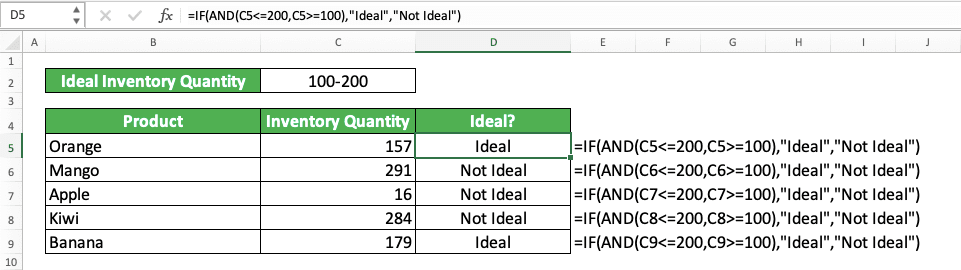In this example, we want to assess whether the inventory quantity we have for our products is already ideal. As the ideal inventory quantity is a number range, we combine IF and AND formulas for the assessment process.

The ideal inventory quantity here is between 100 to 200. Thus, inside our AND, we input logic conditions where our product inventory quantity <= (less than or equal to) 200 and >= (more than or equal to) 100. We put the text “Ideal” if the AND result is TRUE and “Not Ideal” if the AND result is FALSE.

As a result, we get the correct assessment results for all the products’ inventory quantities!

## IF AND then Calculate

You can input various things as the IF true and false results in excel. This includes calculations. If you need to calculate your data depending on your AND result, just input the calculation formulas in your IF.

Here is the general writing form of IF AND with calculations as the IF results.

= IF ( AND ( logic_condition1 , logic_condition2 , … ) , calculation_if_true , calculation_if_false )

You can, of course, just input one calculation as your IF result, whether in its true or false result. It entirely depends on your needs on the IF and AND combination result.

Here is the implementation example of this IF and AND combination concept in excel.We want to add 10 to the score of people who get >80 in test 1 and >70 in test 2. For this, we use IF AND with sum calculation processes as the IF results.

We input the conditions to get the bonus 10 score into our AND inside our IF. After that, we input the total score calculation if a person fulfills the conditions and if he/she doesn’t.

Write the IF AND formula with calculations as its results correctly and we will get the correct result we want!

## IF AND Multiple Conditions

The AND function in excel can hold up to 255 logic conditions as its inputs. Thus, as long as you stay within that number, you can input any multiple logic conditions you need to your AND.

Here is an IF AND implementation example when we input 5 logic conditions to our AND.Just don’t forget to separate each logic condition with a comma sign!

## Nested IF AND in Excel

In a situation where you need to evaluate multiple logic conditions in phases with your IF in excel? If the AND logic suits all your logic conditions evaluation needs, you can nest IF AND to help you here.

Here is the general writing form when you try to nest the IF AND combination in excel.

= IF ( AND ( logic_condition1 , logic_condition2 , … ) , result_if_true , IF ( AND ( logic_condition3 , logic_condition4 , … ) , result_if_true , … , result_if_all_false ) )

In the formula writing, we write the next IF AND as the false result of the previous IF AND. This is so if the previous AND produces FALSE, we will immediately evaluate the logic conditions in the next IF AND. This process, in turn, will create nested IF AND and logic conditions evaluation in phases, just like what we need!

If you use excel 2003 or 2007, then you can nest up to 7 IF AND. If you use excel 2010 or later, however, then you can nest up to 64 IF AND.

Here is the implementation example of this nested IF AND concept in excel.In the example, we try to assess whether each test score group should pass, take a retest, or fail. As we need to evaluate the logic conditions in phases here, we use nested IF AND to do our assessment.

In the first IF AND, we input the logic conditions and result if the test score group passes. Then, we input the logic conditions and result for the test retake in the second IF AND. If all the ANDs we have written produce FALSE, we input a “fail” mark as the nested IF AND result.

We write the correct IF AND for each of test score groups. As a result, we get the assessment results we want as you can see in the example!

## Case Sensitive IF AND

By default, AND isn’t case sensitive when it evaluates the logic conditions that we input into it. If you want to be case-sensitive in the evaluation process, you need to use EXACT inside your AND.

Write EXACT for the logic conditions where you want to compare your data in case sensitive manner. Here is the general writing form when we use EXACT for all the logic conditions in our AND.

= IF ( AND ( EXACT ( data1 , data2 ) , EXACT ( data3 , data4 ) , … ) , result_if_true , result_if_false ) )

EXACT produces TRUE or FALSE depending on whether the data you input into it are similar in values and letter cases. This TRUE or FALSE will mix with other logic conditions results in your AND to produce the final TRUE or FALSE.

Here is the implementation example of the IF AND if we use EXACT in our AND writing. You can see the difference here if we don’t use EXACT in our IF AND.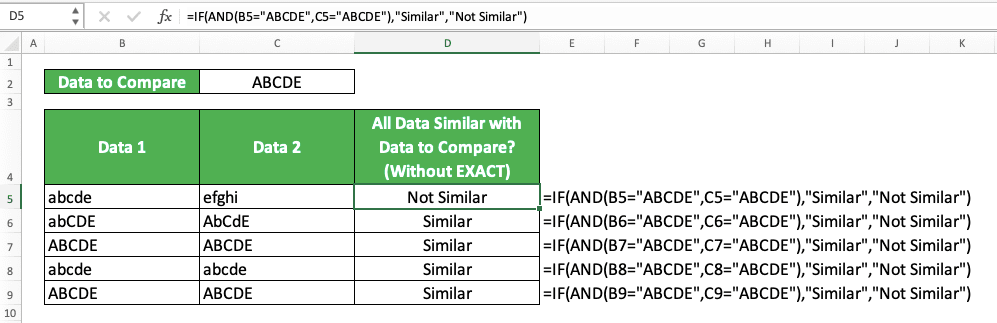As you can see, when we use EXACT, we can be case sensitive when we evaluate data comparison in our AND. If we don’t use the formula, however, then excel will treat different letter cases as similar.

## IF AND OR Statements

You might have known that we can also use OR to combine multiple logic conditions besides AND. The OR logic is different as it will give TRUE if at least one of the logic conditions is TRUE. If all the logic conditions are FALSE, then OR will give us FALSE.

There can be a situation when we need to combine AND and OR to evaluate the logic conditions in our IF. When we have this kind of situation, we can write OR inside AND or AND inside OR depending on our needs.

Here is the general writing form if we write OR inside AND to process the logic conditions in our IF.

= IF ( AND ( OR ( logic_condition1 , logic_condition2 ) , … ) , result_if_true , result_if_false )

And here is the general writing form if we write AND inside OR in our IF.

= IF ( OR ( AND ( logic_condition1 , logic_condition2 ) , … ) , result_if_true , result_if_false )

Combine AND and OR based on your logic conditions evaluation needs so you can get the correct result from your IF!

Here is the implementation example of the IF, AND, and OR combination in excel.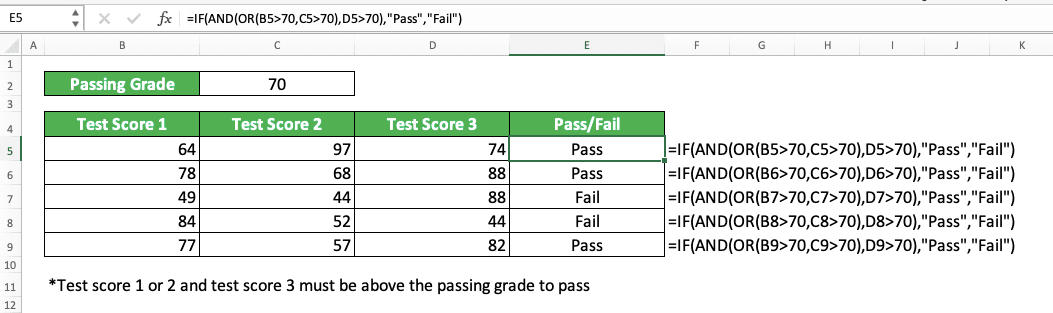In this example, the passing requirement is test 1 or 2 and test 3 score must be above 70. As we need to evaluate logic conditions differently here, we combine AND and OR inside our IF.

We input the passing condition for test 1 and 2 scores inside OR and test 3 score after that inside AND. Then, we input the true and false results of the IF as usual.

As a result of a correct IF, AND, and OR combination, we get the assessment results we need here!

## IF AND Alternative: IF + Multiply Your Logic Conditions

If you don’t want to use AND, you can slip in a star symbol ( * ) in between logic conditions inside your IF. As TRUE is 1 in number and FALSE is 0, this logic conditions multiplication will produce the same result as AND!

Here is the general writing form of the logic conditions multiplication inside your IF.

= IF ( ( logic_condition1 ) * ( logic_condition2 ) … , result_if_true , result_if_false )

And here is its implementation example in excel.Similar results with if you use AND in your IF, right? You can use this method if you need an alternative to the IF and AND combination in excel!

## Exercise

After you have understood how to combine IF and AND formulas in excel completely, now let’s do an exercise. This is so you can become better in your implementation of these two formulas’ combinations.

### Questions

Answer each question in the appropriate gray-colored cells!
1. Passing criteria 1 is scores 1 and 2 must be above 50. What is the passing status of each person? Answer with “Pass” and Fail”!
2. Passing criteria 2 is scores 1 and 3 must be above 60. What is the passing status of each person? Answer with “Pass” and Fail”!
3. Passing criteria 3 is scores 1, 2, and 3 must be above 55. What is the passing status of each person? Answer with “Pass” and Fail”!

You should consider using other functions if you need to nest many IF ANDs to get your result in excel. Too many IF ANDs can make your formula writing look complicated and hard to edit or fix.

For example, something like VLOOKUP can probably replace IF ANDs if you can get the result through a lookup process instead.

Related tutorials you should learn too:

Get updated excel info from Compute Expert by registering your email. It's free!